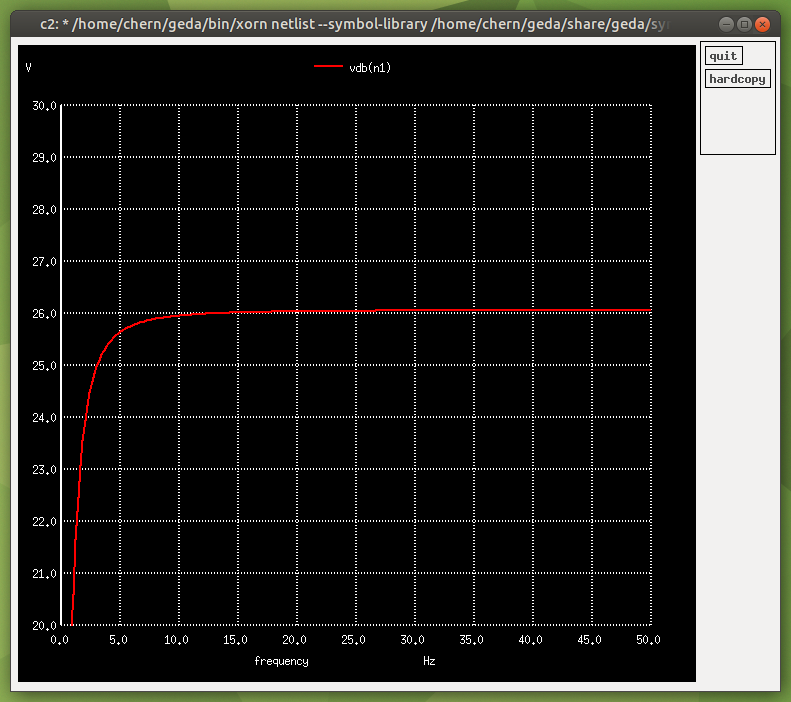# An Introduction To Ngspice

First you'll need to install it, on Debian GNU/Linux the terminal command is:
`sudo apt-get install geda ngspice`
(That's if you have `sudo` installed, if not you can also use `su` to login as root)

This installs ngspice, the circuit simulator, and also gEDA, which includes gschem (a schematic editor) and gnetlist, which we will be using to generate our SPICE netlist.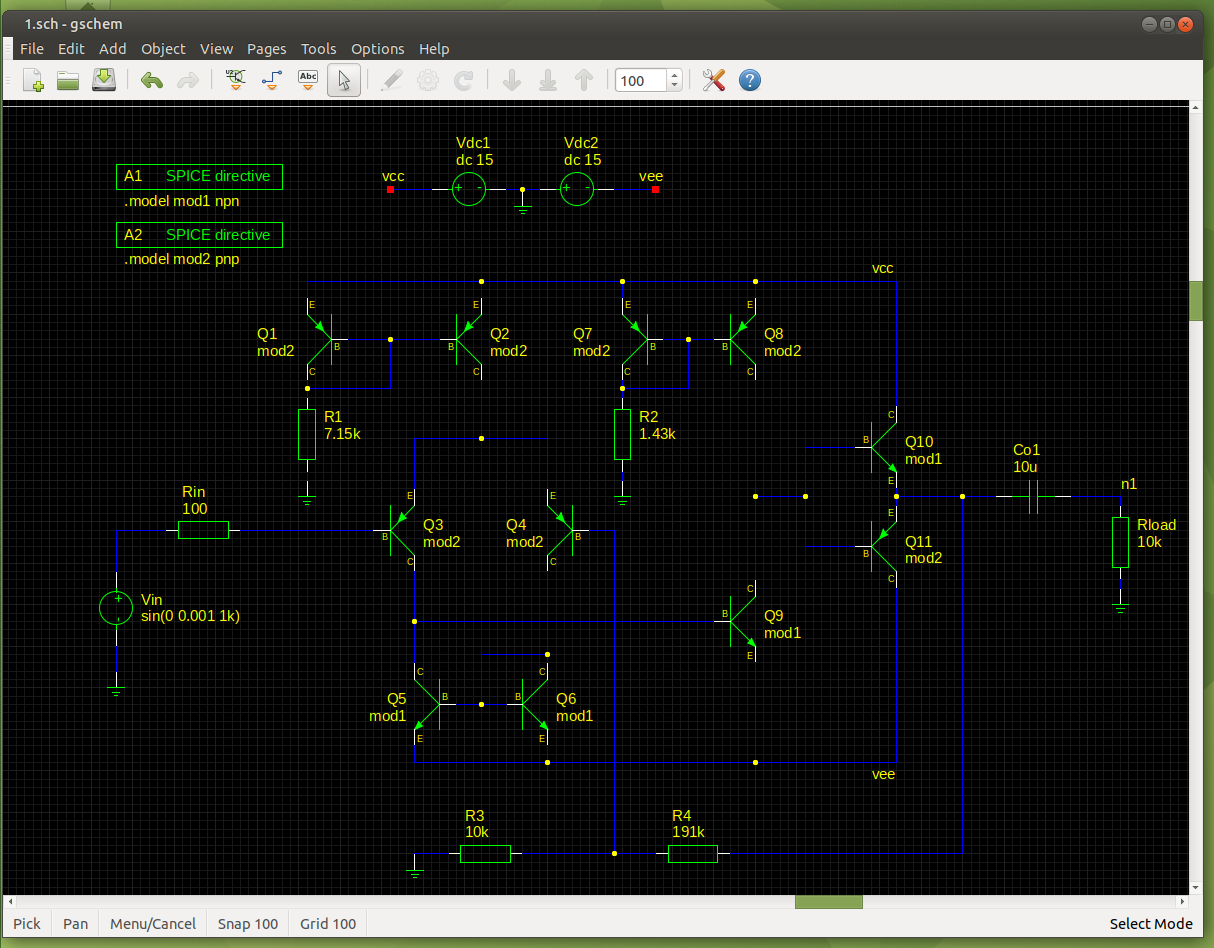For our simulation, we will be using a basic Class B discrete operational amplifier. If you know how to design this circuit, you can skip the next paragraph.

First we decide the supply voltage; I'll be using a ±15VDC split supply. We will assume a load of 10k. Coupling capacitor Co1 forms a high-pass filter with this load, and we do not want it to attenuate any frequencies we want. For audio, the frequency range tends to be 20Hz to 20kHz, so as long as our high-pass filter's cutoff frequency is below 20Hz then it's OK. Let's divide 20Hz by 10 just to be really sure. The value of the capacitor of a capacitive high-pass filter, in Farads, is 1/(2*Pi*fc*R). Pi is approximated at 3.14159, fc (-3dB cutoff frequency) = 2Hz, R = 10kΩ. Round the answer up to the nearest E24 preferred value to get 8.2uF. Let's use a 10uF capacitor. We will assume a source impedance Rin of 100Ω. The input features a PNP Long Tailed Pair (the non-inverting input is the base of transistor Q3 and the inverting input is the base of transistor Q4) and is biased by current from current mirror Q1 Q2. The amount of tail current is 2mA, set by resistor R1: taking into account the Vbe voltage drop of 0.7V, (15V-0.7V)/0.002A = 7.15kΩ (an E192 preferred value). Therefore there is 1mA of current flowing through each collector of this Long Tailed Pair. Current mirror Q5 Q6 acts as an active load. Directly coupled to the inverted output of this Long Tailed Pair is a NPN transistor Q9 that acts as the Voltage Amplification Stage (VAS) in a Common Emitter configuration, and the current through the collector is set to 10mA by resistor R2: (15V-0.7V)/0.01A = 1.43kΩ, and current mirror Q7 Q8 that also acts as an active load. Directly coupled to the collector (output) of transistor Q9 is a Class B Push Pull Output Stage (Q10 Q11). In the non-inverting amplifier configuration, the voltage gain of the amplifier is 1+(R4/R3), about 20 (26dB).

Note that if the previous circuit is capacitively coupled, you will need to provide a resistor to ground (Rb in my schematic) for a DC path for base bias current (the input impedance of the non-inverting input is set to 10kΩ by resistor Rb):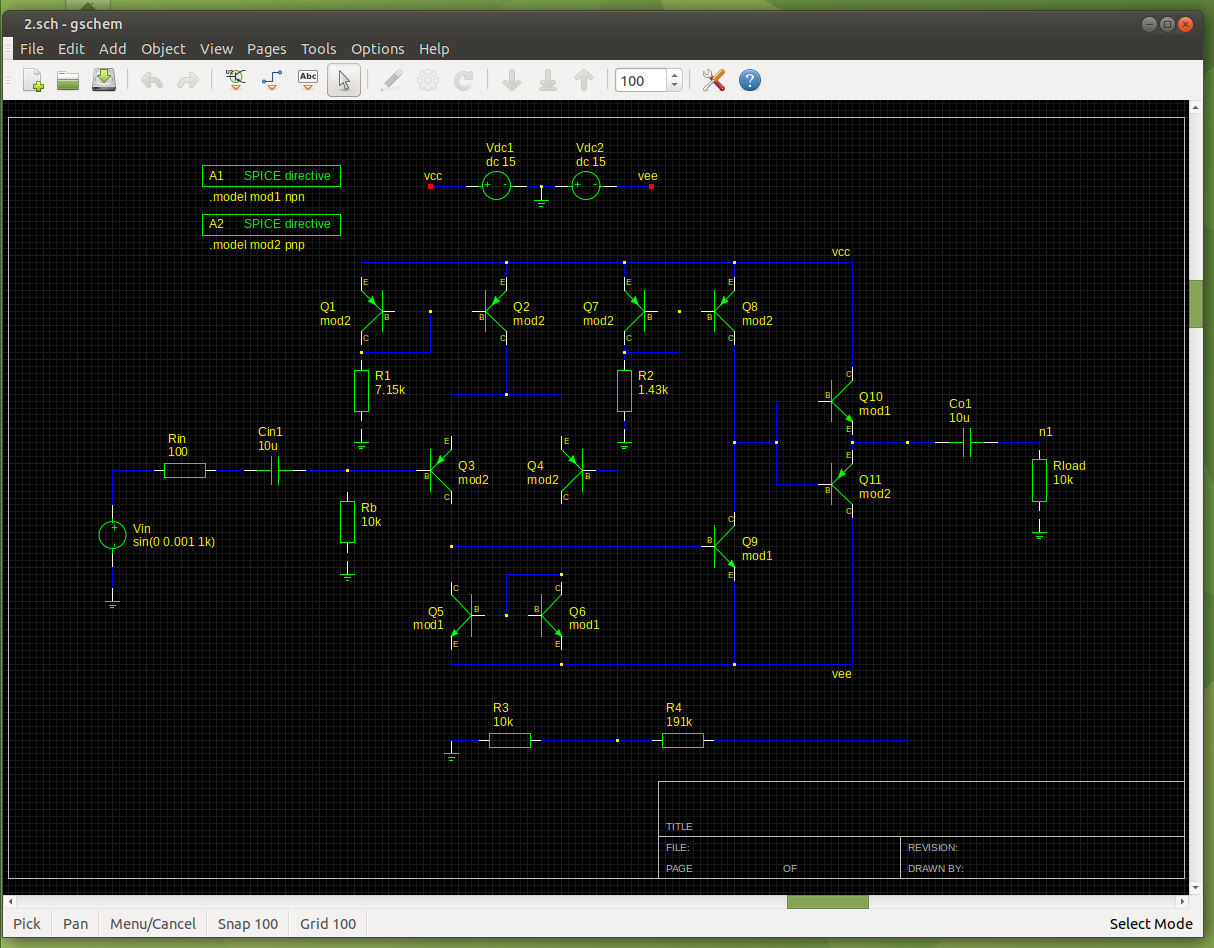Open up gschem. Press i to open up the component library, with this you can search for components to add to the schematic. After adding the component to the schematic, you can click on a component and press e then r to rotate the component. To mirror a component across the y axis, press e then i. Press n to draw connections between component pins. Ctrl+s is to save. Shift+Ctrl+s is to save as. Press ee after clicking on a component or connection (net) to edit the attributes. The refdes is a unique identifier for the component and needs to start with the correct letter followed by an identifier, which can be letters and numbers. For example R5 for a resistor, Cin2 for a capacitor, etc. Under Add Attribute, select "value" from the Name dropdown box and enter the component value. Then click Add to add the value to the component: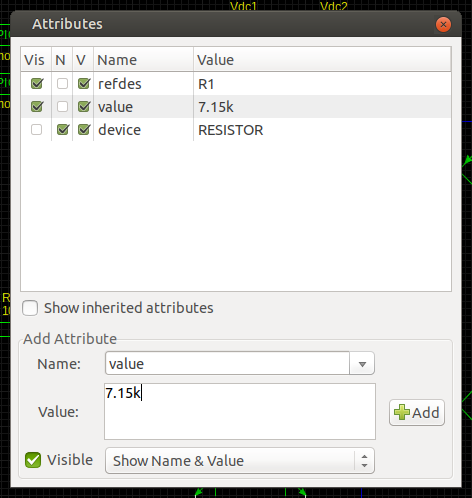In this Edit Attributes window, you can edit values by double clicking them. You can also toggle the visibility of certain parts of the attribute with the check boxes to clean up your schematic.

For the NPN transistor, enter the value "mod1". Then use the Add Component window to insert a SPICE directive (found under SPICE simulation elements). Edit the attributes of this SPICE directive so the value is:
`.model mod1 npn`
`.model` is used to define model parameters, `mod1` is the unique name we give it to use in our schematic. `npn` is the default SPICE model for NPN Type Bipolar Junction Transistors (BJT).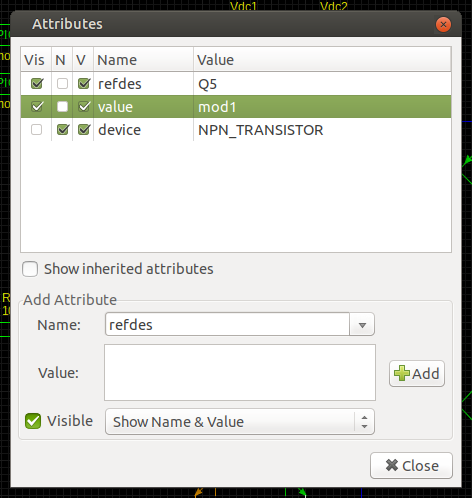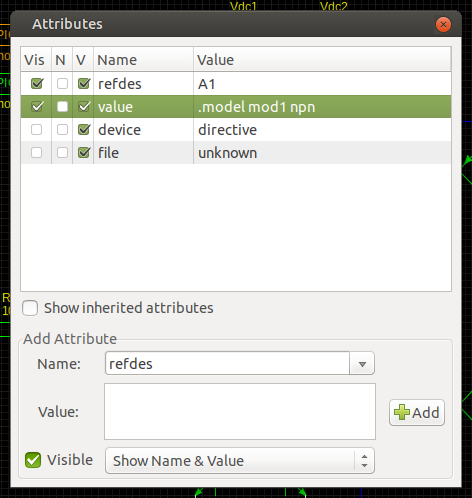Other default SPICE models include:
`pnp` for PNP Type BJT.
`njf` for N-channel Junction Field Effect Transistors (JFET).
`pjf` for P-channel JFET.
`nmos` for N-channel Metal Oxide Semiconductor Field Effect Transistors (MOSFET).
`pmos` for P-channel MOSFET.
`d` for diodes.

Examples of using these models can be found in Volume III of this series of books.

Then you can do the same for the PNP transistors, but using the model name "mod2" and the "pnp" model.

For a 15VDC voltage source, enter the value:
`dc 15`

For a 1mVpeak, 1kHz sine wave, enter:
`sin(0 0.001 1k)`
The first number is the offset in volts, the second number is the peak voltage (in volts), and the third number is the frequency in Hertz. You can also use scientific notation, such as 1E-03 (lower case e will have the same effect as SPICE is not case sensitive).

We will be wanting to measure the voltage across the load resistor Rload, so click on the net and press aa to enter a netname for the test point (I tend to use "n1", "n2", "a1", "output" etc...)

After inputting all the components, editing the refdes and values, and connecting the components, you will end up with something like the schematic at the top of this page. Don't forget to label the nets for the supply rails vcc and vee.

To generate a SPICE netlist, we will be using gnetlist. Assuming a file name of `1.sch`, open up the terminal emulator and (after changing directory to where you saved the schematic) enter:
`gnetlist -g spice-sdb -o 1.net 1.sch`
`-g spice-sdb` tells gnetlist to generate a netlist for the SPICE backend. `-o 1.net` tells gnetlist to name the output file `1.net` and `1.sch` is the name of our input file.
If you have compiled gEDA from source, and gnetlist is in your home folder, the terminal command would be:
`~/geda/bin/gnetlist -g spice-sdb -o 1.net 1.sch`

Now to simulate! Enter:
`ngspice 1.net`
This tells ngspice to open `1.net` for simulation.

First we will be doing a transient simulation, enter:
`tran 0.01m 50m`
This tells ngspice to do a transient simulation with a step of 0.01ms and a stop time of 50ms. You can add a third number for the start time, if not then it will start at zero.
Then:
`plot v(n1)`
This plots the voltage at n1 with reference to ground.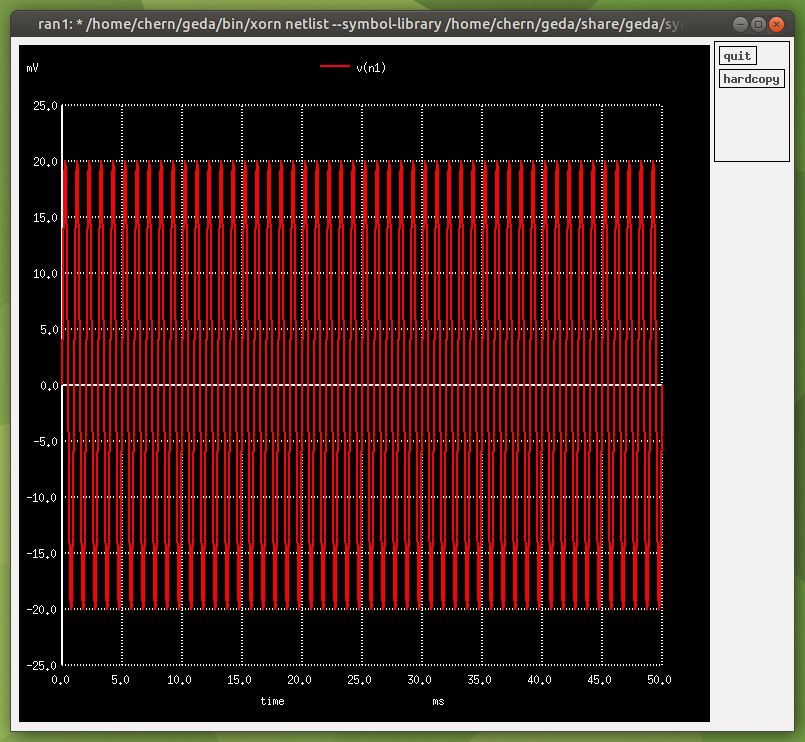Let's see what the current draw through Vdc1 is:
`plot i(vdc1)`
About 23.6mA, not bad! (It appears as a negative number due to a quirk in the way SPICE analyses current).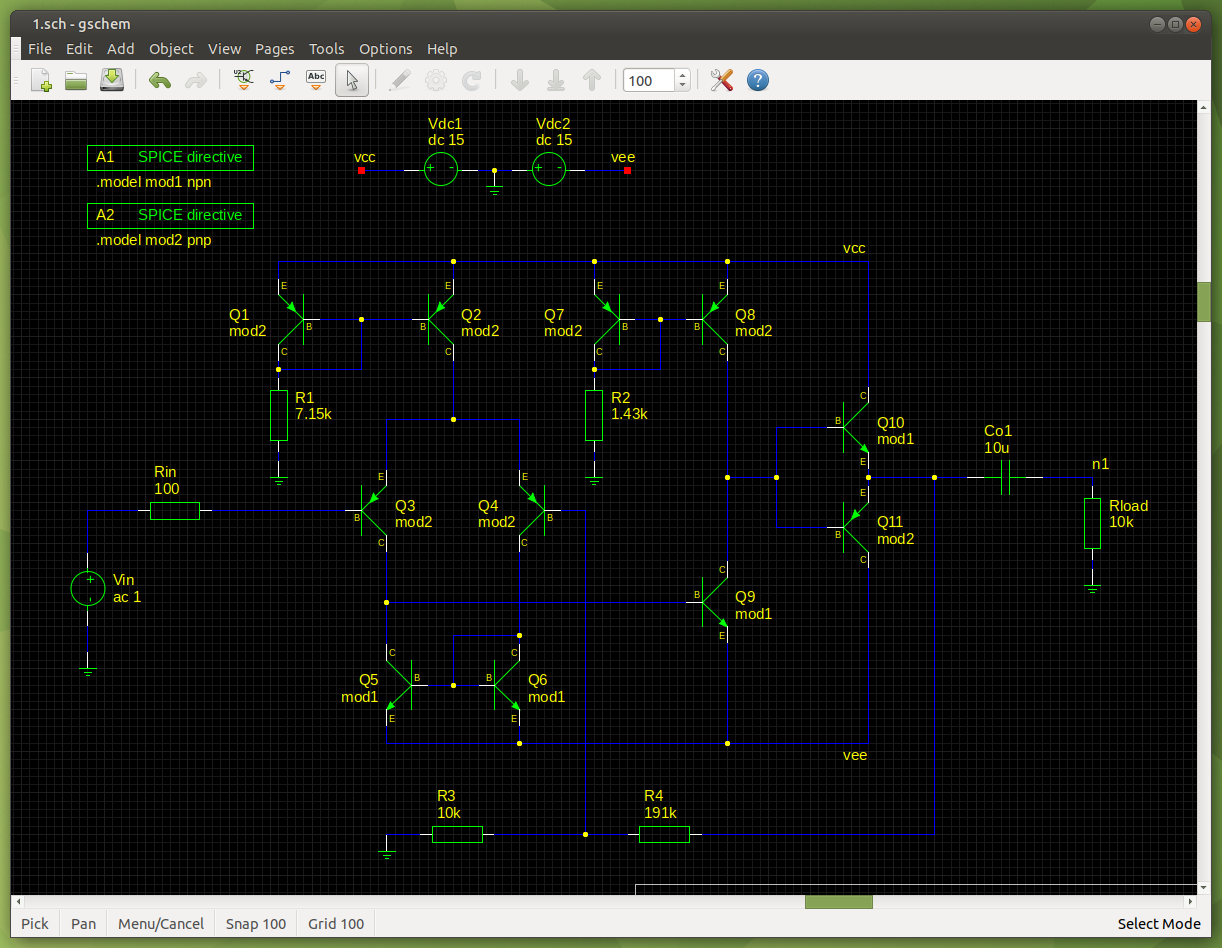Next we will be doing an AC Analysis. Change the value of Vin to `ac 1`, either by editing the schematic and re-exporting the netlist, or by editing the netlist in a text editor.

Then enter:
`ac lin 1000 0.01 30k`
This tells ngspice to do a linear AC Analysis with 1000 steps, starting at 0.01Hz and ending at 30kHz.

Then enter:
`plot vdb(n1)`
This creates a (not strictly) Bode plot of the voltage in decibels (with reference to an AC input of 1) at the point n1 (with reference to ground).
We can see what the voltage gain of our amplifier is by using:
`plot vdb(n1) ylimit 20 30`
`ylimit` limits the y axis of the graph, the first number being the minimum and the second number being the maximum. We can see that the voltage gain of our amplifier is about 26dB!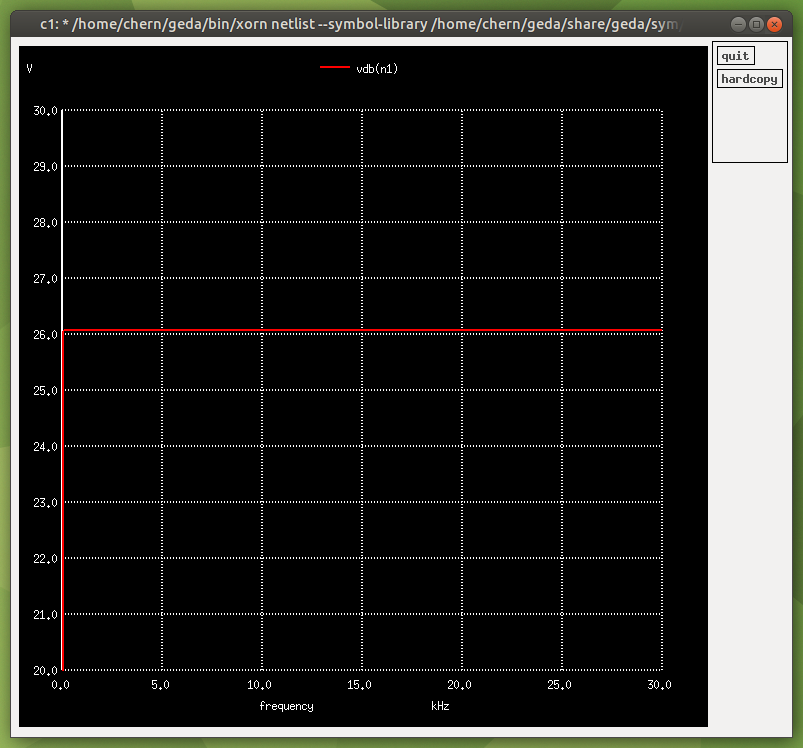Now let's check out the low end!
`ac lin 1000 0.01u 200`
`plot vdb(n1) xlimit 0 50 ylimit 20 30`
`xlimit` limits the x axis of the graph, the first number is the minimum and the second number is the maximum, like `ylimit`.

Sure enough, the frequency response of our amplifier is still flat at 20Hz! You can see that the -3dB cutoff point is less than 2Hz!

If you prefer to use decade or octave scales for the x axis, you can use `dec` or `oct` instead of `lin`.